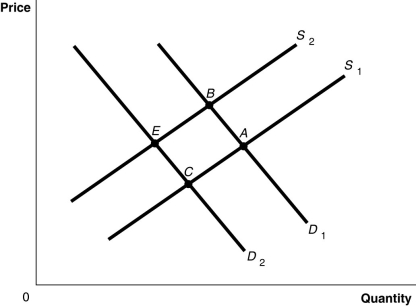# [Solved] Figure 3-8

Question 200
Multiple Choice

## Figure 3-8-Refer to Figure 3-8.The graph in this figure illustrates an initial competitive equilibrium in the market for sugar at the intersection of D₁ and S₂ (point

A)The equilibrium point will move from B to A.
B)The equilibrium point will move from B to C.
B). If there is a decrease in the price of fertilizer used on sugar cane and there is a decrease in tastes for sugar-sweetened soft drinks, how will the equilibrium point change?
C)There will be no change in the equilibrium point.
D)The equilibrium point will move from B to E.

10+ million students use Quizplus to study and prepare for their homework, quizzes and exams through 20m+ questions in 300k quizzes.

Explore our library and get Economics Homework Help with various study sets and a huge amount of quizzes and questions

3.5K

Study sets

66.5K

Quizzes

5.9M

Questions

Upload material to get free accessInvite a friend and get free accessSubscribe and get an instant access